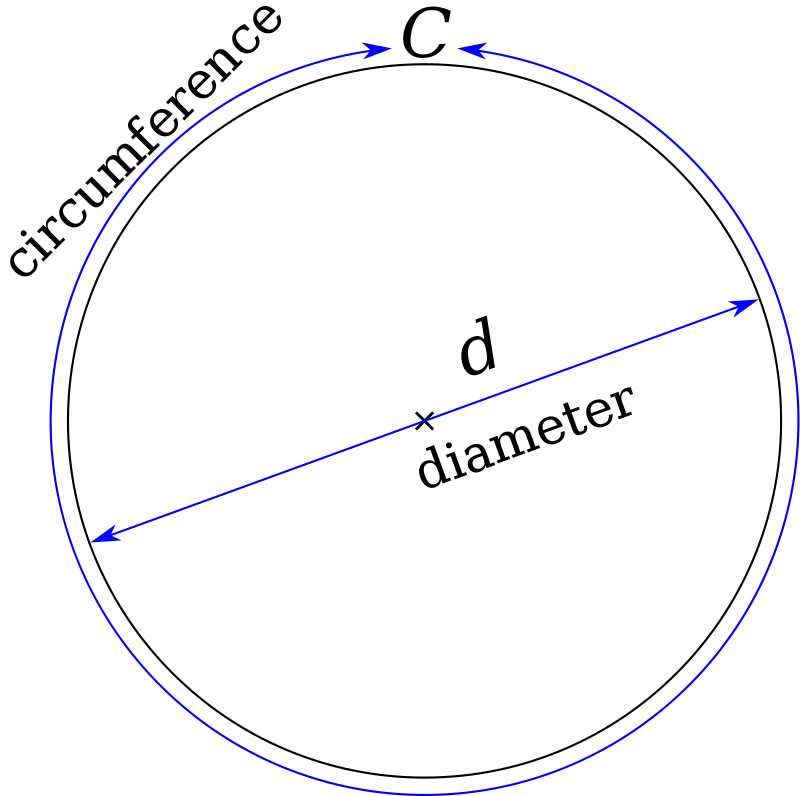# The Pi(π) Machine

(2023) A useless machine.
Black & white spheres, motor, camera, LEDs, Raspberry Pi, custom code.

The Pi(π) Machine is a useless device that calculates the value of Pi (π) by generating 10-bit random numbers using black and white spheres that fall inside a tube. A camera analyses the image and detects the black and white spheres that are translated to 1 and 0 and then to the corresponding number. Using two algorithms, the Monte Carlo method and Euclid’s formula, the machine calculates an approximated value of Pi.

Pi is wonderful precisely because it can only ever be understood theoretically, never actually grasped in its entirety. The lack of solution can be liberating, a demonstration of a classic axiom: The wisest among us know only how little we know.

The greatest mathematical minds of the centuries made advances. Yet every step forward in comprehension and calculation also reveals the limitations of human knowledge. Pi shows that knowing, wholly, is an impossibility.

The more we know, the more apparent it is that there is much more to know. For each individual, and for humanity as a whole, there is always that added bit that simply can’t be figured out, no matter how much information or education we possess. (from https://qz.com/931891/pi-3-14159-is-a-metaphor-for-life-and-the-nature-of-the-universe-no-math-required)

## The MethodPi is the ratio of a circle’s circumference to its diameter.
It cannot be defined as it has an infinite number of decimal digits.
It is approximately equal to 3.14159.

To calculate Pi(π) using the Monte Carlo method, we take pairs of random numbers as x and y and draw them on a 2D plane. Then we count the number of dots m that are within a distance of 1 from the origin, i.e. within the circle as shown below.  The ratio (points within circle)/(total points) will be the approximate ratio of the area of the quarter circle to the area of the square which is π / 4.

## $$\frac{quarter\ circle\ area}{square\ area}= \frac{\frac{pi*r^{2}}{4}}{r^{2}}=\frac{pi*r^{2}}{4 r^{2}}=\frac{pi}{4}$$